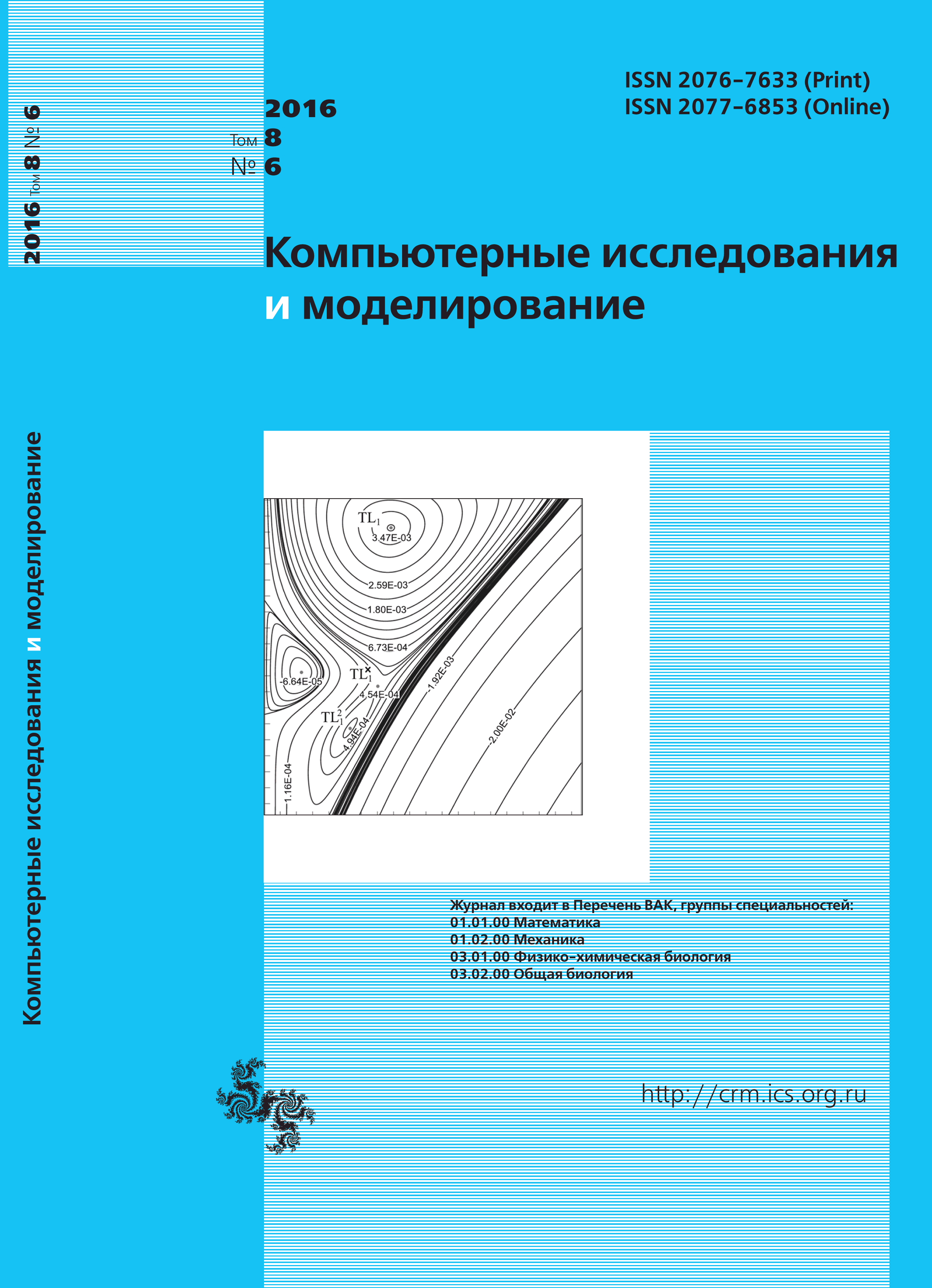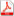Issue 6, 2016 Vol. 8

# All issues

Layered B&eacute;nard–Marangoni convection during heat transfer according to the Newton’s law of coolingpdf (4877K)  / List of references

The paper considers mathematical modeling of layered Benard–Marangoni convection of a viscous incompressible fluid. The fluid moves in an infinitely extended layer. The Oberbeck–Boussinesq system describing layered Benard–Marangoni convection is overdetermined, since the vertical velocity is zero identically. We have a system of five equations to calculate two components of the velocity vector, temperature and pressure (three equations of impulse conservation, the incompressibility equation and the heat equation). A class of exact solutions is proposed for the solvability of the Oberbeck–Boussinesq system. The structure of the proposed solution is such that the incompressibility equation is satisfied identically. Thus, it is possible to eliminate the «extra» equation. The emphasis is on the study of heat exchange on the free layer boundary, which is considered rigid. In the description of thermocapillary convective motion, heat exchange is set according to the Newton’s law of cooling. The application of this heat distribution law leads to the third-kind initial-boundary value problem. It is shown that within the presented class of exact solutions to the Oberbeck–Boussinesq equations the overdetermined initial-boundary value problem is reduced to the Sturm–Liouville problem. Consequently, the hydrodynamic fields are expressed using trigonometric functions (the Fourier basis). A transcendental equation is obtained to determine the eigenvalues of the problem. This equation is solved numerically. The numerical analysis of the solutions of the system of evolutionary and gradient equations describing fluid flow is executed. Hydrodynamic fields are analyzed by a computational experiment. The existence of counterflows in the fluid layer is shown in the study of the boundary value problem. The existence of counterflows is equivalent to the presence of stagnation points in the fluid, and this testifies to the existence of a local extremum of the kinetic energy of the fluid. It has been established that each velocity component cannot have more than one zero value. Thus, the fluid flow is separated into two zones. The tangential stresses have different signs in these zones. Moreover, there is a fluid layer thickness at which the tangential stresses at the liquid layer equal to zero on the lower boundary. This physical effect is possible only for Newtonian fluids. The temperature and pressure fields have the same properties as velocities. All the nonstationary solutions approach the steady state in this case.

Keywords: convection of Benard–Maragoni, analytical solutions, nonstationary problem
Citation in English: Gorshkov A.V., Prosviryakov Y.Y. Layered B&eacute;nard–Marangoni convection during heat transfer according to the Newton’s law of cooling // Computer Research and Modeling, 2016, vol. 8, no. 6, pp. 927-940
Citation in English: Gorshkov A.V., Prosviryakov Y.Y. Layered B&eacute;nard–Marangoni convection during heat transfer according to the Newton’s law of cooling // Computer Research and Modeling, 2016, vol. 8, no. 6, pp. 927-940
DOI: 10.20537/2076-7633-2016-8-6-927-940
• , , . Marangoni effect on the thermal performance of glycerol/water mixture in microchannel. // Applied Thermal Engineering. 2019. — V. 161. — P. 114142.
Please note that citation information may be incomplete as it includes data from Crossref cited-by program partners only.
Views (last year): 10. Citations: 3 (RSCI).

Full-text version of the journal is also available on the web site of the scientific electronic library eLIBRARY.RU

The journal is included in the Russian Science Citation Index

The journal is included in the List of Russian peer-reviewed journals publishing the main research results of PhD and doctoral dissertations.

International Interdisciplinary Conference "Mathematics. Computing. Education"

The journal is included in the RSCI

Indexed in Scopus